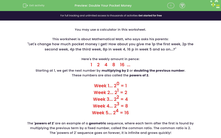# Use the Geometric Sequence of the Powers of 2 to Double Your Pocket Money

In this worksheet, students will use the powers of 2 as a geometric sequence to work out problems involving doubling of pocket money.Key stage:  KS 3

Curriculum topic:   Algebra

Curriculum subtopic:   Use Geometric Sequences

Popular topics:   Algebra worksheets, Money worksheets

Difficulty level:#### Worksheet Overview

You may use a calculator in this activity.

"Let's change how much pocket money I get! How about you give me 1p the first week, 2p the second week, 4p the third week, 8p in week 4, 16p in week 5 and so on...?"

Here's the weekly amount in pence:

1    2    4    8    16  ...

Starting at 1, we get the next number by multiplying by 2 or doubling the previous number.

These numbers are also called the powers of 2.

Week 1... 20 = 1

Week 2... 21 = 2

Week 3... 22 = 4

Week 4... 23 = 8

Week 5... 24 = 16

The 'powers of 2' are an example of a geometric sequence, where each term after the first is found by multiplying the previous term by a fixed number, called the common ratio. The common ratio is 2.

The powers of 2 sequence goes on forever - it is infinite and grows quickly!

Example 1

How much will Matt get, in pence, in week 7?

He will get 26 = 64p.

Example 2

How much will Matt get, in pence, in total by week 4?

He will get 1 + 2 + 4 + 8 = 15p.

Example 3

How much will Matt get, in pounds, in week 17?

He will get 216 = 65,536p = £655.36.

Shall we get started?### What is EdPlace?

We're your National Curriculum aligned online education content provider helping each child succeed in English, maths and science from year 1 to GCSE. With an EdPlace account you’ll be able to track and measure progress, helping each child achieve their best. We build confidence and attainment by personalising each child’s learning at a level that suits them.

Get started